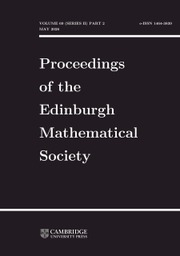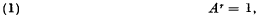Home
Hostname: page-component-99c86f546-kpmwg Total loading time: 0.531 Render date: 2021-12-06T06:52:45.147Z Has data issue: true Feature Flags: { "shouldUseShareProductTool": true, "shouldUseHypothesis": true, "isUnsiloEnabled": true, "metricsAbstractViews": false, "figures": true, "newCiteModal": false, "newCitedByModal": true, "newEcommerce": true, "newUsageEvents": true }Proceedings of the Edinburgh Mathematical Society

# Matrices with finite period

Published online by Cambridge University Press:  20 January 2009

## Extract

HTML view is not available for this content. However, as you have access to this content, a full PDF is available via the ‘Save PDF’ action button.

The study of the primitive solutions of the equationwhere A = (aij) is an n × n matrix whose elements are rational integers, was begun a long time ago. In most cases this equation occurred incidentally in another theory; for instance Jordan encountered it in connection with linear differential equations having algebraic solutions, Minkowski in connection with quadratic forms and Turnbull in geometry. An important fact about these matrices is that any unimodular matrix can be represented as the product of matrices with finite period.

Type
Research Article
Information

## References

1. It will be understood throughout this note that every matrix considered, unless otherwise stated, has all its elements rational integers. Further we shall understand by integer rational integer unless some other meaning is given explicitly.

Among the papers dealing with the problem under consideration are

Baker, H. F., Proc. London Math. Soc. (1), 35 (1903), 379384.Google Scholar

Jordan, C., Journ. f. Math. 84 (1878), 89215, especially 112.Google Scholar

Lipschitz, R., Acta Math. 10 (1887), 137144.CrossRefGoogle Scholar

Minkowski, H., Journ. f. Math. 101 (1887), 196202; also in Gesammelte Abhandlungen 1 (Leipzig-Berlin, 1911), 212218. (Cf. A. Speiser, Theorie der Gruppen von endlicher Ordnung ((3), Berlin, 1937), §67).Google Scholar

Turnbull, H. W., Journ. London Math. Soc. 2 (1927), 242244.CrossRefGoogle Scholar

Vaidyanathaswamy, R., Journ. London Math. Soc. 3 (1928), 121124 and 268272.CrossRefGoogle Scholar

A more complete bibliography is available in the reports of: Wedderburun, J. H. M., Lectures on Matrices (American Math. Soc. Coll. Publications, 17, New York, 1934);Google Scholarvan der Waerden, B. L., Gruppen ron linearen Transformationen (Berlin, 1935); C. C.CrossRefGoogle ScholarMacDuffee, , The Theory of Matrices (Berlin, 1933).CrossRefGoogle Scholar

2. Proofs of the results stated in § 2 will be found in any account of Algebraic Number Theory, e.g. in Hilbert, D., Gesammelte Abhandlungen, 1 (Berlin, 1932), 63–363 or in a forthcoming book by one of us (O. T.): Algebraic Numbers (Oxford, 1940).CrossRefGoogle Scholar

3. It is interesting to observe that it is always possible to find a form of degree n in n variables which is invariant under the transformation determined by an assigned n × n matrix A with | A | = ± 1. To see this denote the latent roots of A by ρ1,......,ρn and denote bya non-trivial solution of the system of equationsThen the formin the n variables u 1, ......, un is easily shown to be invariant under the transformation determined by A.

4. Here and elsewhere we find it convenient to use the summation convention.

5. See e.g. Albert, A. A.Modern Higher Algebra (Chicago-Cambridge, 1937), 37.Google Scholar

6. When we say that A has period r we understand that Ar = 1 and that As ≠ 1 for any s = 1, 2, ......, r − 1.

7. See e.g. Turnbull, H. W. and Aitken, A. C.The Theory of Canonical Matrices (London-Glasgow, 1932), Ch. 5.Google Scholar

8. See e.g. the book of Speiser referred to in 1, §67 or Burnside, W., Proc. London Math. Soc. (2), 7 (1909), 813.CrossRefGoogle Scholar

9. See the papers of Vaidyanathaswamy referred to in 1. The case r=n corresponds to the cyclic permutation of n elements; in this case we have to use the well-known relation10. See the book of Albert referred to in 5, Ch. 4, § 5.

11. Similarly it can be seen that an n x n matrix of period r, whose characteristic polynomial is the power of an irreducible polynomial, must be the direct sum of irreducible matrices each of which has its characteristic function of degree φ(r) Such a matrix can always be obtained as the regular representation of a root of unity contained in an algebraic number field of degree n. CfTaussky, Olga and Todd, John, Proc. Royal Irish Academy, 46A (1940), 111.Google Scholar

12. This result is in accordance with a result established by Higman, , Proc. London Math. Soc. (2), 46 (1940), 231248.CrossRefGoogle Scholar Here it is shown that the only units of finite order in the group-ring of an abelian group are the elements of the group itself.

13. See e.g. the book of Turnbull and Aitken referred to in 7, Ch. 1, §6.You have Access
5
Cited by

# Send article to Kindle

Note you can select to send to either the @free.kindle.com or @kindle.com variations. ‘@free.kindle.com’ emails are free but can only be sent to your device when it is connected to wi-fi. ‘@kindle.com’ emails can be delivered even when you are not connected to wi-fi, but note that service fees apply.

Find out more about the Kindle Personal Document Service.

Matrices with finite period
Available formats
×

# Send article to Dropbox

To send this article to your Dropbox account, please select one or more formats and confirm that you agree to abide by our usage policies. If this is the first time you use this feature, you will be asked to authorise Cambridge Core to connect with your <service> account. Find out more about sending content to Dropbox.

Matrices with finite period
Available formats
×

# Send article to Google Drive

To send this article to your Google Drive account, please select one or more formats and confirm that you agree to abide by our usage policies. If this is the first time you use this feature, you will be asked to authorise Cambridge Core to connect with your <service> account. Find out more about sending content to Google Drive.

Matrices with finite period
Available formats
×
×